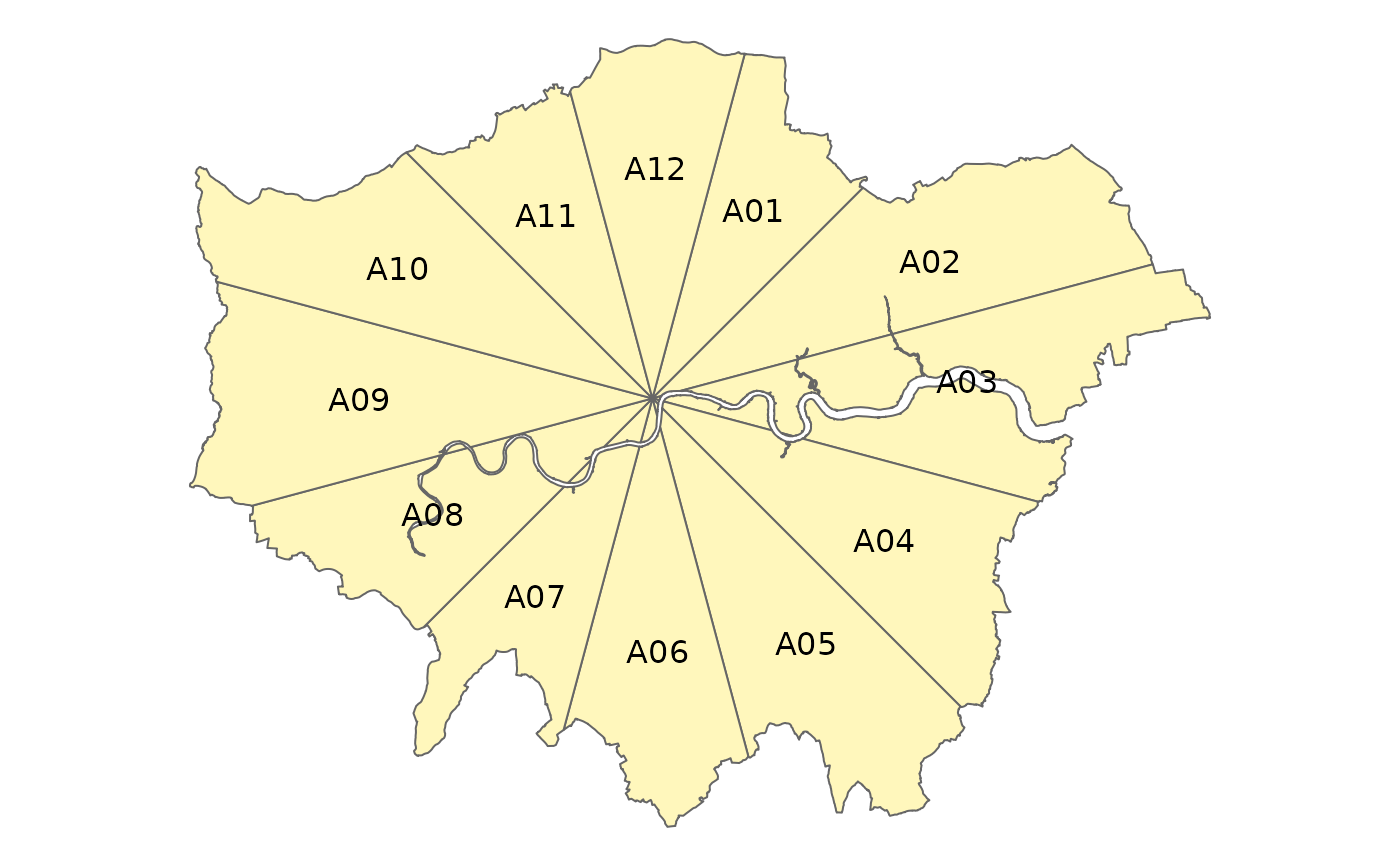Make segments

zb_segment(x = NULL, area = NULL, n_segments = 12, distance = NA)

Arguments

x

Centre point. Should be an sf or sfc object containing one point, or a name of a city (which is looked up with OSM geocoding).

area

(optional) Area. Should be an sf or sfc object containing one (multi) polygon

n_segments

(optional) Number of segments. The number of segments. Either one number which determines the number of segments applied to all circles, or a vector with a number for each circle (which should be a multiple of 4, see also the argument labeling). By default, the central circle is not segmented (see the argument segment_center).

distance

Distance The distances between the circles. For the center circle, it is the distance between the center and the circle. If only one number is specified, distance_growth determines the increment at which the distances grow for the outer circles.

Value

An sf data frame

Examples

zb_plot(zb_segment(london_c(), london_a()))